# Won’t You Be My Neighbor?

K-Nearest Neighbor, step-by-step with scikit-learn

Disclaimer: This write-up is meant as a self-learning guide to understand how functions and algorithms in Machine Learning work. Consult the various references linked throughout this post for more info. The code used for the model described below can be viewed as a Jupyter Notebook file and can be accessed from my GitHub repo.

# Introduction

-Nearest Neighbor (KNN) is a supervised Machine Learningᵀᴹ model for binary classification problems. KNN predicts the class(es) of a value based on the nearest proximity to a K-defined number of neighbors.

Supervised Learning?

Predicting outputs with “new data” using classification or regression models that have been trained with “known” input data

KNN is mainly about two parameters: finding the appropriate number of neighbors (K), and how distance is measured between points. More often than not, KNN is measured by Euclidean distance, the straight-line distance between two points:

Say p refers to a known attribute in our dataset, and q is an unknown attribute that we’re seeking to classify. If we were to plot both p and q on a scatterplot, KNN would define the distance between the two points as the square root of the sum of the squared difference (since we can’t have negative distance) of all attributes (n), for each of the attribute fields (i). Sounds like a mouthful, I know, but all we’re doing is calculating a straight-line distance from an unclassified attribute to the nearest number of neighbors, known attributes, as defined by K:

The unknown attribute will adapt the class of the majority of neighbors within the perimeter of K (so that in the example above, the unknown attribute is classified as B where K=3). Default KNN models have uniform weighting for each attribute’s fields; you can parameterize the model’s by assigning weights for specific attribute features, including distance (if, say, close proximity between points is highly determinant to what its class might be).

But what if the vote is split? For scikit-learn’s KNN function, by default, the attribute will be assigned to the first class in the sequence. So if we have ‘Class 0’ and ‘Class 1’, and we have a tie (say K = 4, and the vote is evenly split), then the attribute will be assigned to ‘Class 0’.

The trick with KNN is defining the appropriate amount of neighbors — K — so that the model’s accuracy is neither underfitting (a ‘complex’ model with not enough neighbors) or overfitting (a ‘simple’ model with too many neighbors) the generalization curve.

Generalization curve?

Describes the accuracy of a model making accurate predictions based on “unseen”, new data. Henceforth, the model is “generalized” from the training to the testing set.

KNN is good introduction to Machine Learning: it is a relatively simple model to understand and interpret, and yields reasonable performance (accuracy) without too many adjustments. It is otherwise known as a lazy learning algorithm. However it doesn’t do well with sparse or voluminous datasets.

# Step 1: Getting Started

cikit-learn, otherwise known as sklearn, is an open-source machine learning library, including different regression and classification models. I recommend checking their vast documentation and tutorials for further insights (instructions to install sklearn here).

Open your preferred python programming environment and import the necessary packages:

`# Generally useful packages to have on deck:import pandas as pdimport numpy as npimport matplotlib.pyplot as plt# If you want to practice with sklearn's prepackaged datasets:from sklearn.datasets import load_wine# The sklearn packages we'll need:from sklearn import metricsfrom sklearn.preprocessing import StandardScalerfrom sklearn.model_selection import train_test_splitfrom sklearn.neighbors import KNeighborsClassifier`

For the sake of practice, sklearn.datasets has all you need to get started. For this particular example however, I fetched some data from Kaggle. Let’s look at a rent dataset for various cities in Brazil:

`df=pd.DataFrame(pd.read_csv('data_practice/houses_to_rent_v2.csv'))display(df.head())df.columns`

# Step 2: Make Sure Your Data is Appropriate for the Model!

otice that in addition to continuous numerical values in the dataset, we have categorical ones as well (city, animal, furniture). Therefore we need to make a handful of adjustments:

a) The fields for ‘animal’ and ‘furniture’ are boolean-like, meaning that they are just indicative whether the unit accepts pets or is furnished (True/False). We can therefore convert the No’s into ‘0s’ and the Yes’s into ‘1s’ (or vice-versa, your call).

b) Well, what about cities? This is where we think about what we want our KNN model to do. There are different ways to look at our classification model: for example, we could simply try and predict whether a rental unit is located in São Paulo (by labeling the city as a ‘1’ and the rest as ‘0’). São Paulo is Brazil and South America’s largest metropolis, so we could make some reasonable assumptions about cost of living reflected in higher rent prices and smaller square footage. But for the purpose of this tutorial, let’s simply experiment with the model to observe how well it fares to predict classification labels for every city in the dataset.

`# converts each unique string values to a unique integerdf.city = pd.factorize(df['city']) # Convert your "boolean" string values into 1s and 0s.df.animal = pd.Series(np.where(df.animal == ‘acept’, 1, 0))df.furniture = pd.Series(np.where(df.furniture == ‘furnished’, 1, 0))`

Also make sure that all your fields are numerical. Especially with datasets that you didn’t have a hand in creating, small adjustments may need to be made.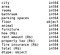Why is the ‘floor’ attribute field type ‘object’ ? 🤔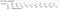Oh, buildings with no floors are labelled as ‘-’, that’s why.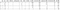I am assigning ‘1’ for ‘True’ and ‘0’ for ‘False’; ultimately the data schema is up to your own discretion.

# Step 3: Scale the dataset

Scaling, also known as standardization, is the process by which data is normalized and replaced with a Z-score: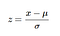x is the sample’s value, μ is the mean and σ is the standard deviation of the training sample (source)

For an explanation on why the data needs standardization, read: Why is scaling required in KNN and K-Means?

`from sklearn.preprocessing import StandardScaler# StandardScaler() transform the data such that its distribution # will have a mean value 0 and standard deviation of 1.scaler = StandardScaler()scaler.fit(X_train)X_train = scaler.transform(X_train)X_test = scaler.transform(X_test)`

StandardScaler() is the function that applies the standardization algorithm, which we chain with the .fit() method to compute the mean and standard deviation of the scaled dataset. Then, we apply the .transform() method to center and scale the distribution of data for both the testing and training sets.

# Step 4: Split the dataset into train and test sets

irst, we need to split the attribute fields from the labels. Meaning, we want to distinguish the predictor variables (X) from the labels we’re seeking to classify (y) for which, in this case, is the ‘city’ attribute field.

`# Excluding ‘city’ and 'total(R\$)', the latter because I think it's # redundant to have a field that's just the sum of 4 other fieldsX = df.iloc[:, 1:-1].values # Including ‘city’ onlyy = df.iloc[:, 0].values`

We now split the dataset into a training and test set: the former is the portion of the dataset that is fed into the model to predict a class, whereas the latter will be used to test the model’s accuracy. Hence what makes KNN a “supervised” classification model.

`X_train, X_test, y_train, y_test = train_test_split(X, y, random_state=0,test_size=0.20)print(X_train.shape, X_test.shape)(8553, 11) (2139, 11)`

Some details about how the train_test_split() function works:

random_state indicates the random sampling method. By default, if not specified, the function will use an instance from numpy.random, and produce a sample that is different if you were to run it multiple times. In this case however, I assigned the random seed to ‘0’. The data is still random, but the method by which the sampling occurs is replicable. I.e. if you were to replicate exactly I did thus far with the same dataset, you’d get the exact same ‘random sample’ if you were to assign the random seed to ‘0’.

test_size is simply the desired proportion of your test set relative to the whole dataset. Thus, at ‘0.20’, I am indicating my test set to be equal to 20% of the entire dataset (from a total of 10,692 rows). By default, the test size is 25%. What’s considered an appropriate size for testing sets depends on the statistical rigor of your model; consult this StackExchange thread for a deeper dive.

# Step 5: Run and interpret the model

sing the KNeighborsClassifier() function, we define the K parameter of the model (just try a random value to start with). We train the KNN model on the training set, and apply it to the testing set with the .predict() method.

`from sklearn.neighbors import KNeighborsClassifier# Set K = 6 (an arbitrary value) to observe the outputknn = KNeighborsClassifier(n_neighbors=6)# Train the algorithmmodel=knn.fit(X_train, y_train)y_pred = model.predict(X_test)print(metrics.accuracy_score(y_test, y_pred))0.6610565684899485`

The model accuracy at K = 6 is approximately 66%. Is that good? Well, it depends on your dataset, research inquiry, what the pertinent background literature in your area of domain expertise says etc.

But we can actually take a deeper look at the accuracy score using sklearn’s classification_report() function.

`from sklearn.metrics import classification_reportprint(classification_report(y_test, y_pred))`

Precision and recall are trade-offs of one another.

Precision: “how many were correctly classified among that class” (source);

Class ‘0’ (standing for São Paulo) has the highest precision, where all instances classified positives were correctly identified 67% of the time.

Recall: “the percentage of total relevant results correctly classified” (source);

For all instances that were classified positive (i.e. instances that correctly belonged to their respective ‘city’ class), Class ‘0’ has a best score of 98%, meaning that the model was effective to identify the relevant elements for that class.

f1-score: “the harmonic mean between precision & recall” (source);

The f1-score is helpful to look at comparatively to the overall accuracy score.

Support: “the number of occurrence of the given class in the dataset” (source);

I have far more occurrences for Class ‘0’ compared to all other classes combined, which may indicate that my dataset isn’t well balanced.

Something else we might to know is the optimal value of K, to yield the best possible prediction accuracy. We can try running the model multiple times, by looping over a range for K:

`# Choose how many neighbors to testk_range = range(1,300)# Create a list to store scoresscores=[]error = []# Run the KNNfor k in k_range:    knn = KNeighborsClassifier(n_neighbors=k)    knn.fit(X_train, y_train)    y_pred = knn.predict(X_test)   # Append the accuracy score    scores.append(metrics.accuracy_score(y_test, y_pred))   # Append the error rate     error.append(np.mean(y_pred != y_test))# Print the scoresprint(scores)`

Inversely, we can try to make a determination about K based on the lowest error rate: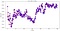Somewhere in the mid-20 range is where K perform best in the model

In this example, a difference of 2% in accuracy isn’t going to meaningfully improve the performance of the model, regardless of the number of neighbors. If anything, this is food for thoughts about what the data is and is not capable of telling us for a classification problem. For example, even if there is a difference in price and area for a mega-city like São Paulo compared to the rest, is the difference really pronounced to make the distinction between comparable-sized cities such as Belo Horizonte and Porto Alegre? And how useful is information about pets allowed or furnished rental to a classification problems about identifying cities?

Sometime the greatest insights gained from a model are the limitations and appropriateness of the dataset itself.

Written by

Written by

## HP-Nunes

#### Aspiring data analyst with a background in GIS. Finishing a Master’s in Environmental Assessment on participatory air monitoring and Citizen Science.